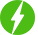# Practical Machine Learning with Scikit-Learn

3

Practical Machine Learning with Scikit-Learn, Learn the most powerful machine learning algorithms in under an hour.

## Course Description

Machine learning is a rapidly growing field. However, a lot of courses on the internet today do not go over some of it’s most powerful algorithms. In this course, we will learn multiple machine learning algorithms, along with data preprocessing, all in under an hour. We will go over regression, classification, component analysis and boosting all in scikit-learn, one of the most popular machine learning libraries for python.

Algorithms we’ll go over (in order):

• Linear Regression
• Polynomial Regression
• Multiple Linear Regression
• Logistic Regression
• Support Vector Machines
• Decision Trees
• Random Forest
• Principle Component Analysis